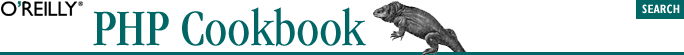home | O'Reilly's CD bookshelfs | FreeBSD | Linux | Cisco | Cisco Exam## 2.10. Formatting Numbers

### 2.10.3. Discussion

The number_format( ) function formats a number by inserting the correct decimal and thousands separators for your locale. If you want to manually specify these values, pass them as the third and fourth parameters:

```\$number = 1234.56;
print number_format(\$number, 2, '@', '#'); // 1#234@56```

The third argument is used as the decimal point and the last separates thousands. If you use these options, you must specify both arguments.

By default, number_format( ) rounds the number to the nearest integer. If you want to preserve the entire number, but you don't know ahead of time how many digits follow the decimal point in your number, use this:

```\$number = 1234.56; // your number
list(\$int, \$dec) = explode('.', \$number);
print number_format(\$number, strlen(\$dec));```